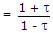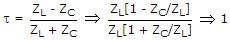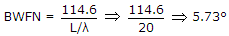# Electronics and Communication Engineering - Electromagnetic Field Theory

31.

The L/C has the dimension of

 A. time B. capacitance C. velocity D. resistance

Explanation:

No answer description available for this question. Let us discuss.

32.

The value of VSWR is taken as ∞ when the line is

 A. short-circuited B. open-circuited C. terminated with full load D. terminated with load equal to the characteristic impedance of the load

Explanation:

For VSWR = ∞ , reflection coefficient must be 1 VSWRif t = 1 VSWR = ∞

for t = 1, ZL = ∞.

33.

The wavelength of an electromagnetic wave after reflection at angle on a surface

 A. remains same as the wavelength perpendicular to the surface B. remains same in the free space C. increases in actual direction of propagation D. decreases in actual direction of propagation

Explanation:

No answer description available for this question. Let us discuss.

34.

A higher value of Standing Wave Ratio indicates

 A. least mismatch between line and load B. greater mismatch between load and line C. number of nodes is equal to the number of antinodes D. none of the above

Explanation:

No answer description available for this question. Let us discuss.

35.

An end fire array consisting of several half-wave lengths of directive gain 40, then Find width of major lobe for broadside

 A. 6° B. 5.73 C. 51.25 D. 0°.